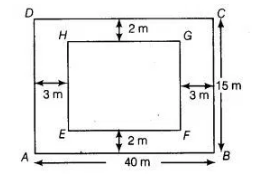# A rectangular plot is given for constructing`
Question:

A rectangular plot is given for constructing a house having a measurement of 40 m long and 15 m in the front. According to the laws, a minimum of 3 m,

wide space should be left in the front and back each and 2 m wide space on each of other sides. Find the largest area where house can be constructed.

Solution:

Let ABCD is a rectangular plot having a measurement of 40 m long and 15 m front.∴ Length of inner-rectangle, EF = 40 – 3 – 3 = 34 m and breadth of inner-rectangle, FG =15 – 2 – 2 = 11 m

∴ Another rectangle EFGH will be formed inside the rectangle ABCD

∴ Area of innerrectangle, EFGH = Length x Breadth

= EF x FG = 34 x 11 = 374 m2

[∴ area of a rectangle = length x breadth]

Hence, the largest area where the house can be constructed in 374 m2.